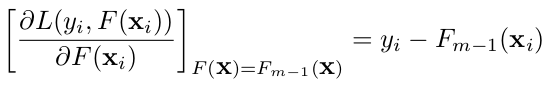# Regression

### Overview

Regression is a technique in statistics and machine learning that attempts to find the relation between one (or more) dependent variable(s) and an independent variable. Imagine graphing features as points on a coordinate plane, then fitting a line to show how the variables influence one another. The benefit of using relational regression is the ability to easily handle high-dimensional attributes without necessarily projecting them into the same space. Furthermore, we can learn the relationship between examples and control whether they are dependent or independent on one another.

The type of regression implemented here is “Least Square Error Tree Boosting (LSTree Boosting),” which learns a series of boosted regression trees and aims to minimize the error of the previous tree.

Loss function: `L(y,F(x)) = (y - F(x))^2`### Preparing Data for Regression

1. Facts

BoostSRL regression cannot explicitly handle continuous features. In order to input facts into BoostSRL, we need to discretize the continuous features using either precomputes or tools like Weka. For example: we discretize the Boston dataset using equal frequency 10-bins on these features: Crim, Indus, Nox, Rm, Age, Dis, Ptratio, B, and Lstat.

``````crim(id143,8).
zn(id257,90).
indus(id124,8).
chas(id12,0).
nox(id314,1).
rm(id131,2).
age(id25,7).
dis(id198,5).
tax(id202,348).
ptratio(id295,1).
b(id50,1).
lstat(id150,5).
...(etc.)
``````
1. Positive Examples

The target predicate medv(exampleid,targetvalue) should be wrapped inside the predicate regressionExample().

For example: the target predicate for the Boston Dataset is medv(exampleid,targetvalue), so the target example will be regressionExample(medv(exampleid),targetvalue).

``````regressionExample(medv(id60),19.6).
regressionExample(medv(id70),20.9).
regressionExample(medv(id63),22.2).
regressionExample(medv(id207),24.4).
regressionExample(medv(id153),15.3).
...(etc.)
``````
1. Negative Examples

There are no negative examples in regression. Because of this, an empty file named `train_neg.txt` should be provided to show that there are none.

``````
``````

### Usage Commands

• Learning:

`java -cp BoostSRL.jar edu.wisc.cs.will.Boosting.Regression.RunBoostedRegressionTrees -reg -l -train train/ -target medv -trees 20`

• Inference:

`java -cp BoostSRL.jar edu.wisc.cs.will.Boosting.Regression.RunBoostedRegressionTrees -i -test test/ -target medv -model train/models/ -trees 20`

Updated: